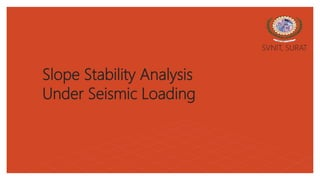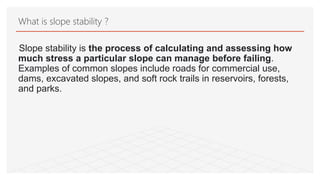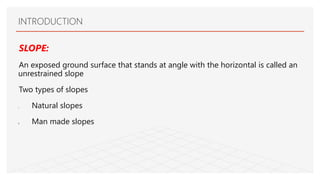Se está descargando tu SlideShare. ×

# Slope Stability Analysis.pptx

Anuncio
Anuncio
Anuncio
Anuncio
Anuncio
Anuncio
Anuncio
Anuncio
Anuncio
Anuncio
AnuncioCargando en…3
×

1 de 20 Anuncio

# Slope Stability Analysis.pptx

This is my seminar presentation on slope stability under seismic loading. if you want report of this seminar then massage me on 8487035203, thank you...

This is my seminar presentation on slope stability under seismic loading. if you want report of this seminar then massage me on 8487035203, thank you...

Anuncio
Anuncio

### Slope Stability Analysis.pptx

2. 2. Slope stability is the process of calculating and assessing how much stress a particular slope can manage before failing. Examples of common slopes include roads for commercial use, dams, excavated slopes, and soft rock trails in reservoirs, forests, and parks. What is slope stability ?
3. 3. SLOPE: An exposed ground surface that stands at angle with the horizontal is called an unrestrained slope Two types of slopes I. Natural slopes II. Man made slopes INTRODUCTION
4. 4. INTRODUCTION Natural slope Man made slope
5. 5. Landslide occurs in both natural and man made slopes Earthquake –induces landslides have caused great amounts of damage and loss of life throughout history Earthquake induced ground shaking is one of the most frequent causes of landslides Thus, evaluation of seismic slope stability is one of the most important activities of earthquake INTRODUCTION
6. 6. Types of slope failures There are a main four types of failures. I. Plain failure II. Rotational failure III. Wedge failure IV. Circular failure
7. 7. Evaluation of slope stability The collection of information on geological, hydrological, topographical, geometrical and material characteristic. Field reconnaissance Monitoring of slope movement. Subsurface investigation. Laboratory test.
8. 8. • The explicit finite element method is used to analyze the seismic slope stability. • Engineers analyze slope to calculate quantitative indicators of performance such as factor of safety, lateral deformation, probability of failure and reliability index. Slope stability analysis includes static and dynamic stability of earth slopes, natural slopes, cut slopes, embankments, landslides, etc. • Limit equilibrium method and slices-based method, which are more traditional methods, are used in the analysis in the stability of soil slopes under seismic loading. Slope stability analysis under seismic load
9. 9. Software used FLAC3D (Fast Lagrangian Analysis of Continua in 3 Dimensions) is numerical modeling software for geotechnical analyses of soil, rock, groundwater, constructs, and ground support.
10. 10. • Different from the LEM and the FE-SRM. • The factor of safety therein is defined as the ratio of projection of vector sum of ultimate resistance forces to that of current driving forces in the potential sliding direction. The VSAM under seismic load
11. 11. The factor of safety can be obtained with the VSAM: 𝑘 = 𝑅 𝑇 𝑅 = 𝑠 (𝜎′𝑠 𝑑)𝑑𝑠 𝑇 = 𝑠 𝜎′ 𝑠 −𝑑 𝑑𝑠 where R and T are the total anti-sliding force and the total sliding force in the potential sliding direction, respectively; and d is the global potential sliding direction. The maximum shear stress can be expressed as 𝜎′𝑇 = −(𝑐 − 𝜎𝑛𝑡𝑎𝑛∅)𝑑𝑡 where c is the cohesion of the soil, φ is the friction angle, and dr is the direction of unit shear stress at any point on the sliding face. The VSAM under seismic load
12. 12. Procedure of seismic stability with VSAM
13. 13. The Effect of Earthquake to Stability and Run Out Distance of Landslide During Rainfall : • a case study of landslide prone area in West Java, Indonesia • Indonesia lies within a convergent zone between three plates, the Pacific plate, the Eurasian plate, and the Australian Indian plate. Case study
14. 14. • Earthquakes are natural disasters characterized by vibrating earth's surface. • Occurrence of landslide. • the movement of a mass of rock, debris, or earth down a slope is called landslide. • The possibility of earthquake induced landslides must also be linked to several factors including topography, geological structure, other landslide causing factors and land use. Case study (introduction)
15. 15. The information is used as the material properties of the avalanche material and the slip fields of the slope model. To determine the prediction of run-out and landslide speed, use numerical simulation of rainfall intensity using Geo- Seep/W, Earthquake Acceleration using Quake/W, and slope stability can be analyzed using Geo-Slope/W Materials and methods
16. 16. Result and discussion
17. 17. The safety factor will decrease with the increasing slope angle as shown in Figure, It shows that under the same soil parameters conditions, the larger the slope angle, the slope conditions will be more unstable. Result and discussion
18. 18. • The intensity of rainfall and earthquake can decrease the value of safety factor. • Factor will decrease along with the enlargement of the slope angle. The larger the angle of the slope, the slope will be more unstable. • As the soil friction angle increases, so does the horizontal displacement of the soil particles applied to seismic loading, but the velocity amplification of the soil particles also decreases as the soil friction angle rises. Conclusion
19. 19. The larger the angle of the slope the greater the thrust caused by the increase in the shear strength of the soil. This is the effect on the run-out, the greater the angle of the slope the lower run-out landslides. The difference of landslide run-out value is caused by the difference in slope, positive water-pore pressure zone and slope shear angle. Case study